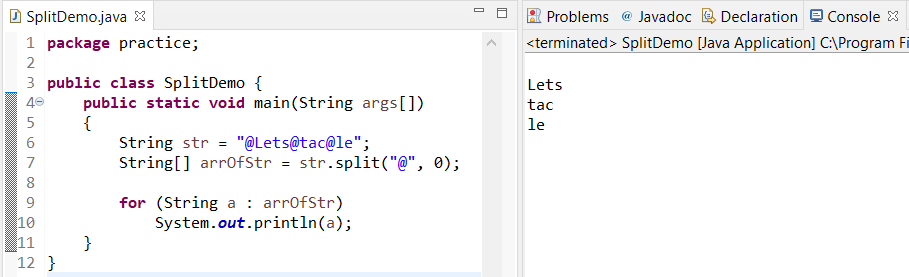How to compare strings in java? Explained with code example and output of the program on string comparison. It covers compare two strings in Java.

To understand how to compare strings in Java, we must understand Strings first. The String is a special class in Java that can contain a character array. It also offers some pre-defined methods for the users to use without knowing anything about the underlying complexity or background details. Some of them are- length(), compare(), concat(), equals(), split(), etc.

### The compare() method

This method compares the 2 strings letter by letter and returns 0 if they match exactly. Otherwise, it returns the ASCII difference of the first pair of mismatched characters. For instance,

``````public class CompareTest {
public static void main(String[] args) {
String a = "A";
String b = "a";
System.out.println("ASCII for A: "+(int)a.charAt(0));
System.out.println("ASCII for a: "+(int)b.charAt(0));
System.out.println("Result: "+a.compareTo(b));
}
}
``````

#### OUTPUTCompareTest.java Output

In the above example, we have stored uppercase ‘A’ in String a and lowercase ‘a’ in String b. When a is compared with b the method returns -32. Which is the ASCII code difference of ‘a’ and ‘A’.

### Using spilt() to Compare Strings in Java

Let’s first understand how the split method works in Java.
Syntax:

``String str_Array[] = str.split("regex","limit"); ``

The method returns a String array which will probably be lesser than the limit provided.

The limit parameter can have three inputs:

Greater than 0: If this is the case then the pattern will be applied at most limit-1 times, the resulting array’s length will not be more than n, and the resulting array’s last entry will contain all input beyond the last matched pattern.
Less than 0: In this case, the pattern will be applied as many times as possible, and the resulting array can be of any size.
Equal to 0: It is similar to a limitless than zero only, trailing empty strings will be discarded.

For instance:

``````public class GFG {
public static void main(String args[])
{
String str = "@Lets@tac@le";
String[] arrOfStr = str.split("@", 2);

for (String a : arrOfStr)
System.out.println(a);
}
} ``````

#### OUTPUTObserving the above example we can easily build a program to count words in a given sentence and thus compare the sentence length. We shall create a method named wordCount where we shall split the given string for every space ” ” character found. Then we have stored it in an array named arr. Which is the iterated counting of each array element which in fact is the word count for the given sentence.

``````
public class SentenceLength {
static int wordCount(String str) {
String arr[] = str.split(" ", 0);
int count = 0;
for(String s: arr) {
count++;
}
return count;
}
public static void main(String[] args) {
String a = "I am a Sentence";
String b = "I am a Sentence too!";
System.out.println("Word count:");
System.out.println("Sentence a - "+wordCount(a));
System.out.println("Sentence b - "+wordCount(b));
}
}
``````

#### OUTPUTJava string word count

### Equals and ‘==’ in Java

Comparing Strings can be tricky! A common program to confuse programmers is given below:

``````public class StringComp {
public static void main(String[] args) {
String s1 = "toto";
String s2 = "toto";
String s3 = new String("toto");
String s4 = new String("toto");
System.out.println("s1 == s2: "+(s1 == s2));
System.out.println("s3 == s4: "+(s3 == s4));
System.out.println("s1 == s3: "+(s1 == s3));
System.out.println("s1.equals(s2): "+(s1.equals(s2)));
System.out.println("s3.equals(s4): "+(s3.equals(s4)));
System.out.println("s1.equals(s3): "+(s1.equals(s3)));
}
}
``````

#### OUTPUTRemove term: how to compare strings in java how to compare strings in java

The output might surprise you if you have seen this problem the first time. When s3 and s4 are compared using the “==” operator or when s1 and s3 are compared using the same the result is false. And that’s because they are comparing the object address stored in the memory which is actually different. As I mentioned earlier String is a special class in Java. We can create objects in two ways:

1. Using ” ” double-quotes
2. Using the new keyword

When a String object is created using double quotes it uses a String pool, which is a part of Java heap space. Here the similar Objects are stored in the same address. But this is not true while using the new keyword. As it does not use String pool for memory allocation to String objects. Therefore every String gets a unique address in this way, in spite of the fact that they can contain the same values.Java Heap String Pool example